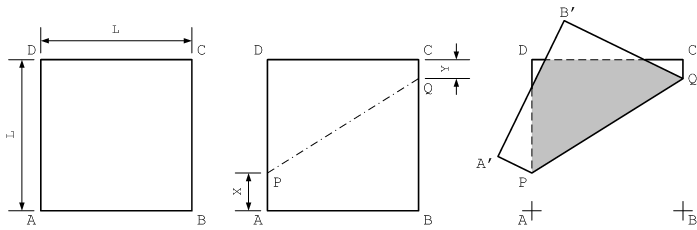# 1222. Area

On mother’s day,the organizer give everyone a square piece of paper, measured L cm in both width and height. Pick a point P on AD with AP = X cm; Pick a point Q on BC with CQ = Y cm. The paper is then folded along PQ.

Partychen what you to write a program to help him to calculate the area of overlap (the grey region) as shown in the figure.### 输入格式

The first line of the input is an integer N (0<N<5000). Each of the following N lines contains three non-negative real numbers L, X, Y separated by space.

You may assume that L is always positive and that X, Y ≤ L.

### 输出格式

Write a line for each input containing the area of overlap. Numbers should be rounded to 3 digits after the decimal point.

### 样例

Input
3
100.0 50.0 50.0
100.0 0.0 0.0
250.0 50.0 80.0

Output
5000.000
5000.000
25230.314


0 人解决，5 人已尝试。

0 份提交通过，共有 13 份提交。

9.9 EMB 奖励。